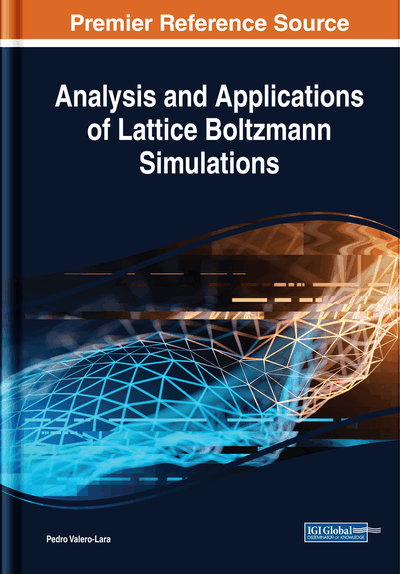# Lattice Boltzmann Shallow Water Simulation With Surface Pressure

Iñaki Zabala (SENER Ingeniería y Sistemas S.A., Spain) and Jesús M. Blanco (Universidad del País Vasco, Spain)
DOI: 10.4018/978-1-5225-4760-0.ch009
Available
\$37.50
No Current Special Offers

## Abstract

Shallow water conditions are produced in coastal and river areas and allow the simplification of fluid solving by integrating in height to the fluid equations, discarding vertical flow so a 3D problem is solved with a set of 2D equations. Usually the boundary conditions defined by the surface pressure are discarded, as it is considered that the difference in atmospheric pressure in simulation domain is irrelevant in most hydraulic and coastal engineering scenarios. However, anticyclones and depressions produce noticeable pressure gradients that may affect the consequences of phenomena like tides and tsunamis. This chapter demonstrates how to remove this weakness from the LBM-SW by incorporating pressure into the LBM for this kind of scenario in a consistent manner. Other small-scale effects like buoyancy may be solved using this approach.
Chapter Preview
Top

## Governing Equations

The conservation of mass (Ghia, et al., 1982). at a volume elementis represented by the continuity equation:

(1) with velocity components u, v and w.

This represents that the rate of change of mass in a volume element is equal to the mass flow into the volume and the variation of mass due to change on density.

For an incompressible flow densityis constant so this simplifies to:

(2)

Using vector notation and the nabla operator, these equations in a general coordinate system read:

(3)

The conservation of momentum at a volume elementis represented by the following momentum equations:

(4)

They consider the rate of change of momentum in such a volume element that is the momentum flux (Bouzidi et al., 2001), into the volume plus the shear and normal stresses acting on the volume element plus the forces acting on the mass of the volume.

The forces acting on the volume mass include the gravity and coriolis as well as the electric and magnetic forces that act on a flow, and are denoted byThe pressure p can be written as the trace of the stress tensor:

(5)

The minus sign takes into account the fact that the pressure acts as a negative normal stress.

(6)(7)

For Newtonian fluids the following relations hold:

(8)

And with the symmetry condition:

(9)

Inserting the normal stresses and shear stresses according to Equation (8) into the conservation of momentum equations Equation (7), we obtain the equations:

(10)

For incompressible flows, we can use the continuity equationto obtain:

(11)

Using the continuity equation Equation (11), and assuming constant viscosity these may be rewritten in nonconservative form:

(12) or, in more compact Einstein notation:

(13)

## Complete Chapter List

Search this Book:
Reset